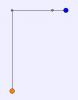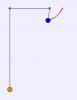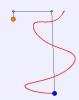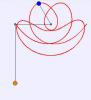BrainDen.com - Brain Teasers

## Question

J  -- -- -- --o
|
|
|
|
|
|
o

Attached at ends of a one unit long and weightless cord  are two identical balls with a unit mass.
If the cord slides on hook J w/out friction.. How far can the higher ball can be pulled
horizontally away  from J  ,so that when released it can hit  the lower ball?
•1

## Recommended Posts

• 0

I assumed a constant tension on the string.

Looking at conservation of energy is simpler, but naively applying it gives an initial sideways distance of 1/2.

That would mean the lower weight would fall, at first, then rise back to its initial height.

Is that the right answer?

##### Share on other sites

• 0

If I modeled it correctly,

The higher ball will have fallen 84.44% of its horizontal distance when its string reaches vertical.

So if it is pulled a distance of .5921 from the hook it will fall a distance of .5 when it reaches vertical.

The balls will then collide.

Nice puzzle.

##### Share on other sites

• 0

A little more fun:

Let the lower mass be a fraction f of the upper mass.

What are the values of f for which the upper ball should be pulled distances of

[a] 0.5 and 1.0 from the hook in order for the balls to collide?

•1
##### Share on other sites

• 0

If I modeled it correctly,

The higher ball will have fallen 84.44% of its horizontal distance when its string reaches vertical.

So if it is pulled a distance of .5921 from the hook it will fall a distance of .5 when it reaches vertical.

The balls will then collide.

Nice puzzle.

Is the lower ball at rest when they collide?

##### Share on other sites

• 0

Is the lower ball at rest when they collide?

No. Since the upper ball ends up closer to the hook than when it started, the lower ball is moving downward.

##### Share on other sites

• 0

Is the lower ball at rest when they collide?

No. Since the upper ball ends up closer to the hook than when it started, the lower ball is moving downward.

Yes, the lower ball moves downward..but  it decelerate to  v=0 as the opposing up pull cause by the swinging ball becomes equal to

its weight upon impact (both vertical)..So the raised lower ball start from v=0 to v=max to v=0.  Unless with experiments, I'm unsure

Since  potential energy = kinetic energy of the system

J
|  |
|  |
|  |
|  |
|  |
|  |
|  |
|  |
|  |
|  |
oo 1/2L     initial energy =0

J --- --- --- --- --- --- --- --- --- ---o Ep=mg(1/2)
|
|
|
o                                                   Ep=mg(h)
h
--            raised energies: mg(1/2+h) = 1/2m V^2 (swinging ball)

...a perpetual motion

##### Share on other sites

• 0

I will recheck my model. Momentum comes into play.

If we assume the string is not sliding in the hook at impact, then the lower ball is stationary.

But we can't just assume it, we somehow have to derive it.

##### Share on other sites

• 0

I assumed a constant tension on the string.

Looking at conservation of energy is simpler, but naively applying it gives an initial sideways distance of 1/2.

That would mean the lower weight would fall, at first, then rise back to its initial height.

Is that the right answer?

Maybe you are right. And I  don't rely much on the ff:

a) the swinging ball is 1/2+h away from when released.
b) its distance from the hook always > 1/2 before impact.
c) it has no vertical velocity on impact
d) it follows a curve non radial or radial but on different center
e) the sliding ball  move downward only
f) the tension on string is lowest at the instant of release
g)the given values on the problem are complete

I post the same problem on a science forum (classic physics section) just to comfirm a thing. A member claimed to have done an experiment where the lower ball remain at rest until  collision and that is to prove the earlier post of a moderator. But I doubt if there  had been an experiment, and still hoping for the calculated values.

Yes , a nice puzzle bonanova..thanks

##### Share on other sites

• 0

A very, very cool puzzle, indeed.

This arrangement of weights and pulleys is known as the Atwood Machine.

When one of the weights is allowed to swing in pendulum fashion it's called the Swinging Atwood Machine.

Its analysis is difficult, and yields best to Lagrangian methods.

The motion of the swinging weight is governed by the tension in the string, gravity,

and a centrifugal force of varying magnitude. The path can be chaotic or periodic, and its

shape changes qualitatively depending on the ratio of the hanging and swinging weights.

The swinging weight acquires an initial downward momentum during the part of its path

when its fall is unopposed by the force of the hanging weight (when the cord is horizontal)

When the mass ratio is unity, the force of the hanging weight never overcomes this

downward momentum, with the result that the swinging weight continues to descend

until the hanging weight rises, and eventually hits the pulley.

For the equal-weight case, the length of the cord of the swinging weigh decreases, initially, owing

to the initial tension in the cord. Ir reaches its minimum length of 79.76% at an angle of 54 degrees.

As it acquires velocity, however its centrifugal force overcomes the tension, and its cord length

increases. At vertical, it has lengthened to 87% of its original length. Because of its continuing

downward momentum, its path is only approximately horizontal at this point: it has a small

downward component.

For a unit-length string, the weights will collide if the swinging weight has initial cord length of .5/.87 = 0.574.

As it continues swinging, its cord length continually increases, until the hanging weight

eventually rises at hits its pulley, after which the swinging weight becomes a simple pendulum.

If the hanging weight is only slightly heavier, however, it will eventually overcome the downward

momentum of the swinging weight, which, while still swinging, will begin to rise, eventually to

attain its original height. If this occurs at an extremity of its swinging motion, it will retrace its

path, and the motion will be periodic. In general this does not happen and the motion is aperiodic.

I used my simulation model to verify the case for equal weights that the swinging weight

has a slight downward component at vertical. Then I did some searching on the web and

eventually found a delightful java program that plots things for various input parameters.

That model uses two pulleys, just so the hanging weight does not interfere with the swinging weight.

Here is the start: the ratio of the hanging weight to the swinging weight is 1.0When the weight reaches vertical the motion is nearly horizontal, but with a slight downward component.

The cord length has increase to 87% of initial length.Later, it's still falling.Eventually the hanging weight hits the hook.Now, slightly increase the ratio of the hanging to swinging weight to 1.119.

After 3 1/2 swings, it hits its lowest point.It then rises, and comes back to horizontal, but on the opposite side of the hookIt then falls back, retraces its path, and continues in periodic motion.

Now make the weight ratio = 1.2

The weight does not fall as far before its downward momentum is reversed.And for this ratio, the motion is aperiodic.

This is after about 14 oscillations.Now make the weight ratio = 2.4

To expand the figure, I made the swinging cord length twice as long.

The heavier hanging weight creates enough centrifugal force to launch the swinging weight

over the top, creating a really weird, but very pleasant, smiling face.And, for this ratio, the motion is periodic.

## Join the conversation

You can post now and register later. If you have an account, sign in now to post with your account.×   Pasted as rich text.   Paste as plain text instead

Only 75 emoji are allowed.

×   Your previous content has been restored.   Clear editor

×   You cannot paste images directly. Upload or insert images from URL.